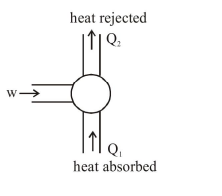# If minimum possible work is done by a refrigerator

Question:

If minimum possible work is done by a refrigerator in converting 100 grams of water at $0^{\circ} \mathrm{C}$ to ice, how much heat (in calories) is released to the surrounding at temperature $27^{\circ} \mathrm{C}$ (Latent heat of ice $=80 \mathrm{Cal} /$ gram to the nearest integer?

Solution:$w+Q_{1}=Q_{2}$

$w=Q_{2}-Q_{1}$

C.O.P. $=\frac{Q_{1}}{w}=\frac{Q_{1}}{Q_{2}-Q_{1}}=\frac{273}{300-273}=\frac{Q_{1}}{W}$

$\mathrm{w}=\frac{27}{273} \times 80 \times 100 \times 4.2$

$\mathrm{Q}_{2}=\mathrm{w}+\theta_{1}$

$\mathrm{Q}_{2}=\frac{27}{273} \times 80 \times 100 \times 4.2+80 \times 100 \times 4.2$

$\mathrm{Q}_{2}=\frac{300}{273} \times 80 \times 100=8791.2 \mathrm{cal}$